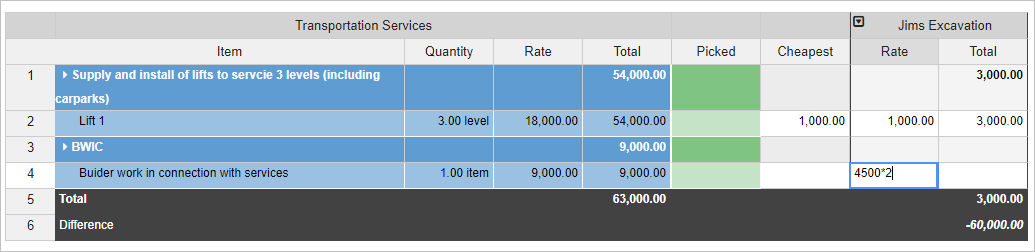Formula in Bartender

Entering Formula in Bartender

Mathematical symbols such as Addition (+), Subtraction (-), Multiplication (*), Division (/) and Percentage (%) are now available to perform simple calculations or a combination of calculations on the Quotes page.

Calculations which follow the BODMAS rule (Brackets, Orders. Division, Multiplication, Addition, Subtraction) can be used in the Item Rate or Total cells, Heading Total and Quote Total.

You can click on an Item rate, Item total, Quote Heading or Quote Total in a Quote to enter a formula.Fig 1. Entering Formula for an Item Rate

0 out of 0 found this helpful